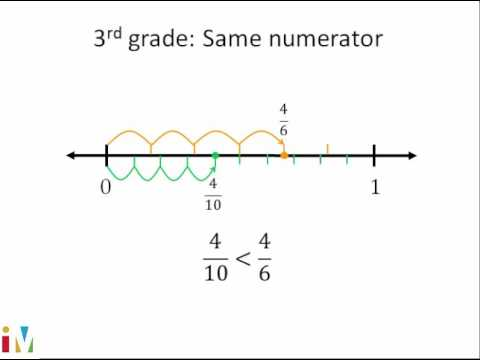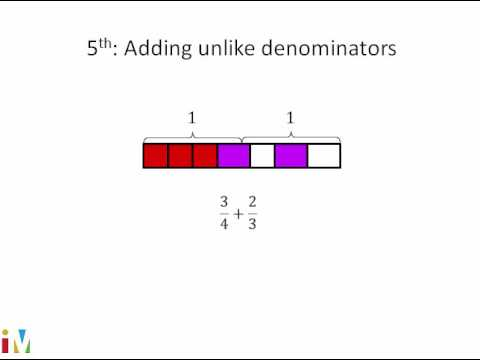Video

# Dividing a unit fraction by a whole number (Full video)

Description: This video uses an area model to explain 1/3 ÷ 5. So let's see if we can figure out what 1/3 divided by five is. Try to draw out 1/3 of a whole and then divide it into five equal sections. And now let me split up into three equal sections.

### Other videos you might be interested in### Comparing Fractions

#### Illustrative Mathematics